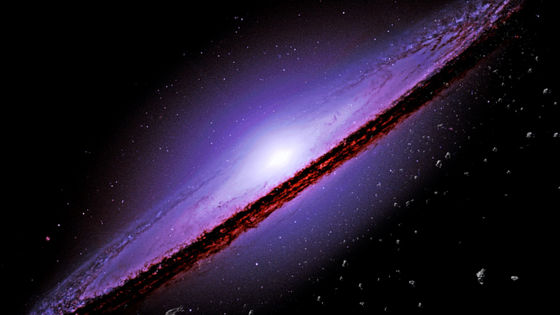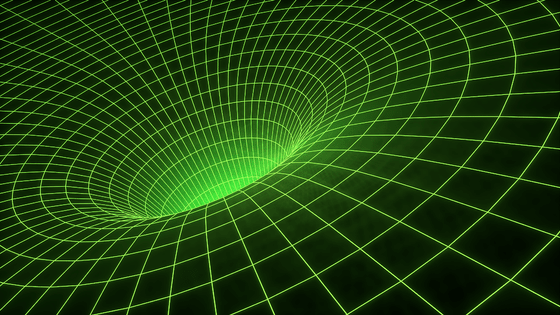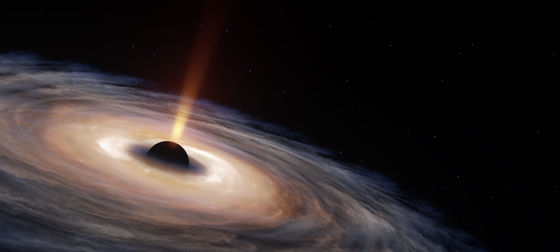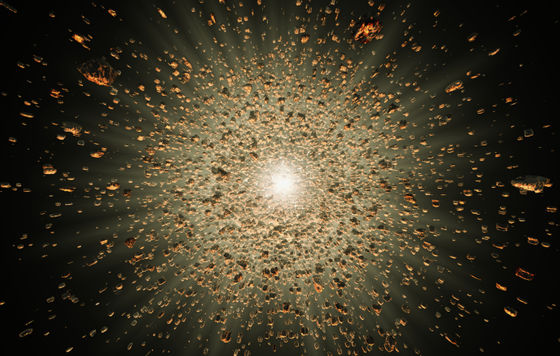What is the 'singularity' of a black hole or the beginning of the universe?There are 'singularities ' in the universe that cannot be explained by existing theories. Live Science, a science news site, explained singularities that appear in topics related to astronomy and black holes, but often do not know exactly what they really are.

What is a singularity? | Live Science
https://www.livescience.com/what-is-singularity

◆ What is the 'singularity' in the first place?
◆ Black hole 'gravitational singularity'
◆ Cosmic censorship hypothesis and 'naked singularity'
◆ Was there a 'singularity' in front of the Big Bang?

◆ What is the 'singularity' in the first place?
'Singularity' is a term that appears in research fields such as artificial intelligence and the humanities, but when physicists use it when discussing the universe, 'the formula used to understand the universe is Refers to 'a place that malfunctions'.

For example, if the value of X becomes zero in an equation containing '1 / X', the value of the equation becomes infinite and the theory does not hold. Usually, the theory doesn't hold when something is missing in the equation, or when it's physically impossible, but physics has a singularity that can't be cleared up. There are several. The most famous of these is the ' gravitational singularity ' where the gravitational field becomes infinite.◆ Black hole 'gravitational singularity'

The singularity of gravity appears in Einstein's general theory of relativity, which is said to be 'the theory that best describes gravity to date.'

Karl Schwartzschild, a physicist who discovered a black hole based on the general theory of relativity, first applied the general theory of relativity to a simple celestial body with a spherical mass like a normal star. Then, the calculation result showed that there is a singular point, that is, a place where the general theory of relativity does not hold, at a position separated by the Schwarzschild radius from the center of the celestial body.This singularity has long been controversial as it could break the general theory of relativity, but as research progresses, 'it's okay if the radius of the star is larger than the radius of Schwarzschild.' understand. For example, the Schwarzschild radius of the sun is 3 km, while the radius of the sun is about 700,000 km, so the sun can be fully explained by the general theory of relativity. This singularity is called a 'coordinate singularity' because it does not become a singularity in some places.

However, the problem was not solved when the radius of the star was less than the Schwarzschild radius. Moreover, when a real celestial body is compressed so strongly that it becomes smaller than the Schwarzschild radius, it becomes a black hole, and at the center of it, the object is crushed to an infinitely small point by strong gravity. This point is the 'true singularity' and is sometimes called the 'gravitational singularity' because gravity becomes infinite.

◆ Cosmic censorship hypothesis and 'naked singularity'
If the general theory of relativity is broken because the problem of singularity cannot be solved, it is not the case. Because nothing comes out from the inside of the black hole where even light cannot escape, and the inside of the black hole cannot be observed. It has nothing to do with. 'The boundary surface that separates the inside and outside of a black hole in this way is called the

event horizon. Also, the idea that the singularity is always beyond the event horizon was named the ' cosmic censorship hypothesis ' because it looks as if someone is hiding the singularity.

However, recent theoretical studies and computer simulations have pointed out the possibility that there may be singularities that are exposed without being protected by the event horizon, that is, 'naked singularities.' Researchers are also divided on whether or not there is a truly naked singularity.

◆ Was there a 'singularity' in front of the Big Bang?
From the general theory of relativity and the results of actual observations of the universe, it is known that the universe has continued to expand since the Big Bang occurred about 13.77 billion years ago. Therefore, if we rewind the time by about 13.77 billion years, we will reach the infinitely small point, that is, the singularity, which existed before the universe expanded. However, as mentioned above, the general theory of relativity does not work at singularities.To solve this problem, researchers are looking for a quantum gravity theory that goes beyond the general theory of relativity, and one of the candidates is the causal set theory .

This theory of causal sets thinks that the universe is made up of endless causal relationships, so something must have happened before something happened. In other words, since the universe has been around for an infinite number of times, it means that the universe had no beginning, and the problem of the singularity that existed before the Big Bang is solved. The specific theory is summarized in the following article.

What is the new theory that the universe continued infinitely before the Big Bang, pointing out that 'the universe never started'? --GIGAZINE

in Science, Posted by log1l_ks